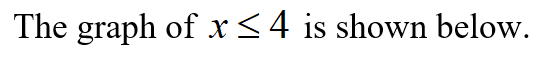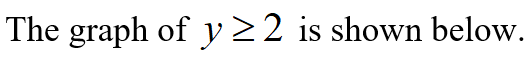# An objective function and a system of linear inequalities representing constraints are given. Complete parts​ (a) through​ (c). Objective Functionzequals=3 x plus y3x+yConstraintsleft brace Start 3 By 1 Matrix 1st Row 1st Column x less than or equals 4 2nd Row 1st Column y greater than or equals 2 3rd Row 1st Column 4 x minus y greater than or equals minus 2 EndMatrixx≤4y≥24x−y≥−2a. Graph the system of inequalities representing the constraints.Graph each inequality individually and then use those results to graph the system. Combining these three inequalities results in the graph shown to the right.b.Find the value of the objective function at each corner of the graphed region. Now calculate the value.c. Use the values in part​ (b) to determine the maximum value of the objective function and the values of x and y for which the maximum occurs.

Question
31 views
An objective function and a system of linear inequalities representing constraints are given. Complete parts​ (a) through​ (c).

Objective Function
zequals=3 x plus y3x+y
Constraints
left brace Start 3 By 1 Matrix 1st Row 1st Column x less than or equals 4 2nd Row 1st Column y greater than or equals 2 3rd Row 1st Column 4 x minus y greater than or equals minus 2 EndMatrix
 x≤4 y≥2 4x−y≥−2
a. Graph the system of inequalities representing the constraints.
Graph each inequality individually and then use those results to graph the system. Combining these three inequalities results in the graph shown to the right.
b.Find the value of the objective function at each corner of the graphed region. Now calculate the value.
c. Use the values in part​ (b) to determine the maximum value of the objective function and the values of x and y for which the maximum occurs.
check_circle

Step 1

a

It is given that the objective function is z = 3x + y.

Constraints are:

Step 2Step 3...

### Want to see the full answer?

See Solution

#### Want to see this answer and more?

Solutions are written by subject experts who are available 24/7. Questions are typically answered within 1 hour.*

See Solution
*Response times may vary by subject and question.
Tagged in

### Math